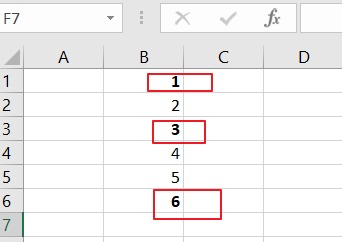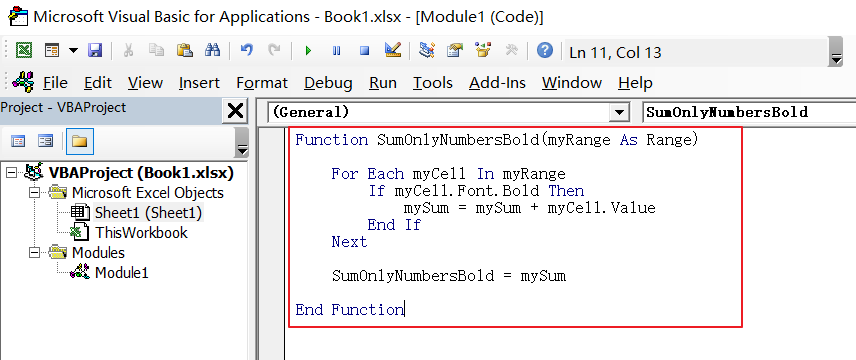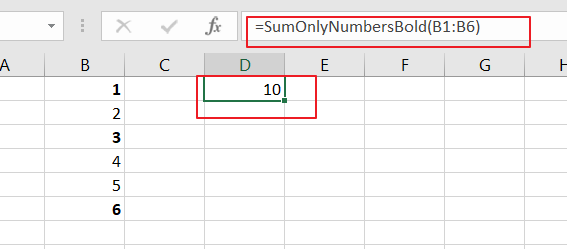# How to Sum Only Numbers in Bold in a Range of Cells in Excel

This post will guide you how to sum only numbers with bold style in a range of cells in Excel. How do I sum on cells with bold font within a range of cells using User defined function in Excel 2013/2016.

## Sum Bold Numbers

Assuming that you have a list of data in range B1:B6, which contain some bold or normal numbers. and you wish to sum only numbers with bold font style, how to quickly sum those number based on bold font style in the given range of cells in Excel. And this tuotrial will show you how to sum only the bold numbers with a User Defined Function in your worksheet. Just do the following steps:Step1# open your excel workbook and then click on “Visual Basic” command under DEVELOPER Tab, or just press “ALT+F11” shortcut.Step2#  then the “Visual Basic Editor” window will appear.

Step3# click “Insert” ->”Module” to create a new module.Step4# paste the below VBA code into the code window. Then clicking “Save” button.```Function SumOnlyNumbersBold(myRange As Range)

For Each myCell In myRange
If myCell.Font.Bold Then
mySum = mySum + myCell.Value
End If
Next

SumOnlyNumbersBold = mySum

End Function```

Step5# back to the current worksheet, then type the following formula in a blank cell , and then press Enter key.

`=SumOnlyNumbersBold(B1:B6)`Sidebar